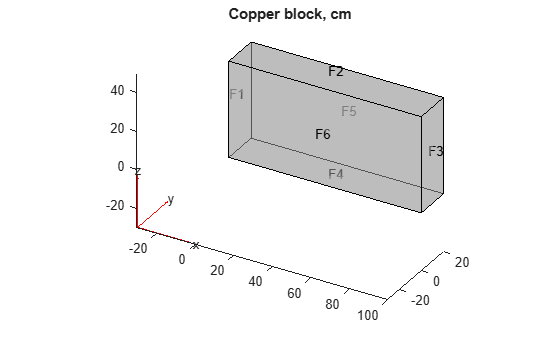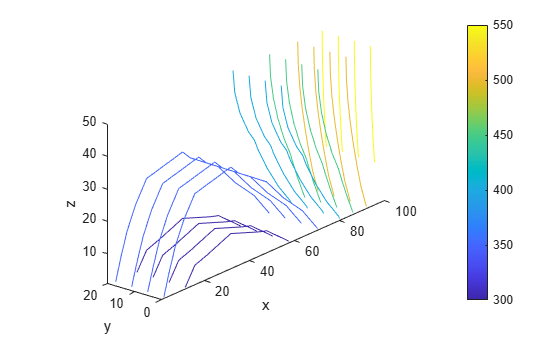# interpolateTemperature

Interpolate temperature in a thermal result at arbitrary spatial locations

## Syntax

``Tintrp = interpolateTemperature(thermalresults,xq,yq)``
``Tintrp = interpolateTemperature(thermalresults,xq,yq,zq)``
``Tintrp = interpolateTemperature(thermalresults,querypoints)``
``Tintrp = interpolateTemperature(___,iT)``

## Description

example

````Tintrp = interpolateTemperature(thermalresults,xq,yq)` returns the interpolated temperature values at the 2-D points specified in `xq` and `yq`. This syntax is valid for both the steady-state and transient thermal models.```

example

````Tintrp = interpolateTemperature(thermalresults,xq,yq,zq)` returns the interpolated temperature values at the 3-D points specified in `xq`, `yq`, and `zq`. This syntax is valid for both the steady-state and transient thermal models.```

example

````Tintrp = interpolateTemperature(thermalresults,querypoints)` returns the interpolated temperature values at the points in `querypoints`. This syntax is valid for both the steady-state and transient thermal models.```

example

````Tintrp = interpolateTemperature(___,iT)` returns the interpolated temperature values for the transient thermal model at times `iT`.```

## Examples

collapse all

Create a thermal model for steady-state analysis.

`thermalmodel = createpde('thermal');`

Create the geometry and include it in the model.

```R1 = [3,4,-1,1,1,-1,1,1,-1,-1]'; g = decsg(R1, 'R1', ('R1')'); geometryFromEdges(thermalmodel,g); pdegplot(thermalmodel,'EdgeLabels','on') xlim([-1.5,1.5]) axis equal```Assuming that this is an iron plate, assign a thermal conductivity of 79.5 W/(m*K). Because this is a steady-state model, you do not need to assign mass density or specific heat values.

`thermalProperties(thermalmodel,'ThermalConductivity',79.5,'Face',1);`

Apply a constant temperature of 300 K to the bottom of the plate (edge 3). Also, assume that the top of the plate (edge 1) is insulated, and apply convection on the two sides of the plate (edges 2 and 4).

```thermalBC(thermalmodel,'Edge',3,'Temperature',300); thermalBC(thermalmodel,'Edge',1,'HeatFlux',0); thermalBC(thermalmodel,'Edge',[2,4],... 'ConvectionCoefficient',25,... 'AmbientTemperature',50);```

Mesh the geometry and solve the problem.

```generateMesh(thermalmodel); results = solve(thermalmodel)```
```results = SteadyStateThermalResults with properties: Temperature: [1541x1 double] XGradients: [1541x1 double] YGradients: [1541x1 double] ZGradients: [] Mesh: [1x1 FEMesh] ```

The solver finds the values of temperatures and temperature gradients at the nodal locations. To access these values, use `results.Temperature`, `results.XGradients`, and so on. For example, plot the temperatures at nodal locations.

```figure; pdeplot(thermalmodel,'XYData',results.Temperature,... 'Contour','on','ColorMap','hot');```Interpolate the resulting temperatures to a grid covering the central portion of the geometry, for `x` and `y` from `-0.5` to `0.5`.

```v = linspace(-0.5,0.5,11); [X,Y] = meshgrid(v); Tintrp = interpolateTemperature(results,X,Y);```

Reshape the `Tintrp` vector and plot the resulting temperatures.

```Tintrp = reshape(Tintrp,size(X)); figure contourf(X,Y,Tintrp) colormap(hot) colorbar```Alternatively, you can specify the grid by using a matrix of query points.

```querypoints = [X(:),Y(:)]'; Tintrp = interpolateTemperature(results,querypoints);```

Create a thermal model for steady-state analysis.

`thermalmodel = createpde('thermal');`

Create the following 3-D geometry and include it in the model.

```importGeometry(thermalmodel,'Block.stl'); pdegplot(thermalmodel,'FaceLabels','on','FaceAlpha',0.5) title('Copper block, cm') axis equal```Assuming that this is a copper block, the thermal conductivity of the block is approximately 4 W/(cm*K).

`thermalProperties(thermalmodel,'ThermalConductivity',4);`

Apply a constant temperature of 373 K to the left side of the block (edge 1) and a constant temperature of 573 K at the right side of the block.

```thermalBC(thermalmodel,'Face',1,'Temperature',373); thermalBC(thermalmodel,'Face',3,'Temperature',573);```

Apply a heat flux boundary condition to the bottom of the block.

`thermalBC(thermalmodel,'Face',4,'HeatFlux',-20);`

Mesh the geometry and solve the problem.

```generateMesh(thermalmodel); thermalresults = solve(thermalmodel)```
```thermalresults = SteadyStateThermalResults with properties: Temperature: [12691x1 double] XGradients: [12691x1 double] YGradients: [12691x1 double] ZGradients: [12691x1 double] Mesh: [1x1 FEMesh] ```

The solver finds the values of temperatures and temperature gradients at the nodal locations. To access these values, use `results.Temperature`, `results.XGradients`, and so on. For example, plot temperatures at nodal locations.

```figure; pdeplot3D(thermalmodel,'ColorMapData',thermalresults.Temperature)```Create a grid specified by `x`, `y`, and `z` coordinates and interpolate temperatures to the grid.

```[X,Y,Z] = meshgrid(1:16:100,1:6:20,1:7:50); Tintrp = interpolateTemperature(thermalresults,X,Y,Z);```

Create a contour slice plot for fixed values of the `y` coordinate.

```figure Tintrp = reshape(Tintrp,size(X)); contourslice(X,Y,Z,Tintrp,[],1:6:20,[]) xlabel('x') ylabel('y') zlabel('z') xlim([1,100]) ylim([1,20]) zlim([1,50]) axis equal view(-50,22) colorbar```Alternatively, you can specify the grid by using a matrix of query points.

```querypoints = [X(:),Y(:),Z(:)]'; Tintrp = interpolateTemperature(thermalresults,querypoints);```

Create a contour slice plot for four fixed values of the `z` coordinate.

```figure Tintrp = reshape(Tintrp,size(X)); contourslice(X,Y,Z,Tintrp,[],[],1:7:50) xlabel('x') ylabel('y') zlabel('z') xlim([1,100]) ylim([1,20]) zlim([1,50]) axis equal view(-50,22) colorbar```Solve a 2-D transient heat transfer problem on a square domain and compute temperatures at the convective boundary.

Create a transient thermal model for this problem.

`thermalmodel = createpde('thermal','transient');`

Create the geometry and include it in the model.

```g = @squareg; geometryFromEdges(thermalmodel,g); pdegplot(thermalmodel,'EdgeLabels','on') xlim([-1.2,1.2]) ylim([-1.2,1.2]) axis equal```Assign the following thermal properties:

• Thermal conductivity is 100 W/(m*C)

• Mass density is 7800 kg/m^3

• Specific heat is 500 J/(kg*C)

```thermalProperties(thermalmodel,'ThermalConductivity',100,... 'MassDensity',7800,... 'SpecificHeat',500);```

Apply insulated boundary conditions on three edges and the free convection boundary condition on the right edge.

```thermalBC(thermalmodel,'Edge',[1,3,4],'HeatFlux',0); thermalBC(thermalmodel,'Edge',2,... 'ConvectionCoefficient',5000,... 'AmbientTemperature',25);```

Set the initial conditions: uniform room temperature across domain and higher temperature on the left edge.

```thermalIC(thermalmodel,25); thermalIC(thermalmodel,100,'Edge',4);```

Generate a mesh and solve the problem using `0:1000:200000` as a vector of times.

```generateMesh(thermalmodel); tlist = 0:1000:200000; thermalresults = solve(thermalmodel,tlist);```

Define a line at convection boundary and compute temperature gradients across that line.

```X = -1:0.1:1; Y = ones(size(X)); Tintrp = interpolateTemperature(thermalresults,X,Y,1:length(tlist));```

Plot the interpolated temperature `Tintrp` along the `x` axis for the following values from the time interval `tlist`.

```figure t = [51:50:201]; for i = t p(i) = plot(X,Tintrp(:,i),'DisplayName', ... strcat('t=',num2str(tlist(i)))); hold on end legend(p(t)) xlabel('x') ylabel('Tintrp')```## Input Arguments

collapse all

Solution of thermal problem, specified as a `SteadyStateThermalResults` object or a `TransientThermalResults` object. Create `thermalresults` using `solve`.

Example: ```thermalresults = solve(thermalmodel)```

x-coordinate query points, specified as a real array. `interpolateTemperature` evaluates temperatures at the 2-D coordinate points `[xq(i),yq(i)]` or at the 3-D coordinate points `[xq(i),yq(i),zq(i)]`. So `xq`, `yq`, and (if present) `zq` must have the same number of entries.

`interpolateTemperature` converts query points to column vectors `xq(:)`, `yq(:)`, and (if present) `zq(:)`. It returns temperatures in the form of a column vector of the same size. To ensure that the dimensions of the returned solution is consistent with the dimensions of the original query points, use `reshape`. For example, use ```Tintrp = reshape(Tintrp,size(xq))```.

Data Types: `double`

y-coordinate query points, specified as a real array. `interpolateTemperature` evaluates temperatures at the 2-D coordinate points `[xq(i),yq(i)]` or at the 3-D coordinate points `[xq(i),yq(i),zq(i)]`. So `xq`, `yq`, and (if present) `zq` must have the same number of entries. Internally, `interpolateTemperature` converts query points to the column vector `yq(:)`.

Data Types: `double`

z-coordinate query points, specified as a real array. `interpolateTemperature` evaluates temperatures at the 3-D coordinate points `[xq(i),yq(i),zq(i)]`. So `xq`, `yq`, and `zq` must have the same number of entries. Internally, `interpolateTemperature` converts query points to the column vector `zq(:)`.

Data Types: `double`

Query points, specified as a real matrix with either two rows for 2-D geometry, or three rows for 3-D geometry. `interpolateTemperature` evaluates temperatures at the coordinate points `querypoints(:,i)`, so each column of `querypoints` contains exactly one 2-D or 3-D query point.

Example: For 2-D geometry, ```querypoints = [0.5,0.5,0.75,0.75; 1,2,0,0.5]```

Data Types: `double`

Time indices, specified as a vector of positive integers. Each entry in `iT` specifies a time index.

Example: `iT = 1:5:21` specifies every fifth time-step up to 21.

Data Types: `double`

## Output Arguments

collapse all

Temperatures at query points, returned as an array. For query points that are outside the geometry, `Tintrp` = `NaN`.